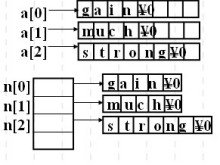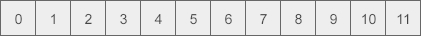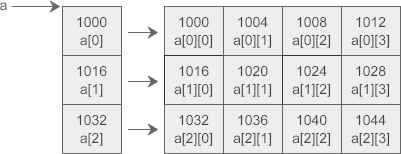# C++定义指针数组，数组指针，指针数据

https://www.cnblogs.com/warmfrog/p/3695173.html

C语言或C++中，数组元素全为指针的数组称为指针数组

eg: int*ptr_array;

### 与二维数组对比char a={"gain","much","strong"};

char *n={"gain","much","strong"};

3×8的空间，而给n分配的空间则取决于具体字符串的长度。

http://c.biancheng.net/view/2022.html

int a = { {0, 1, 2, 3}, {4, 5, 6, 7}, {8, 9, 10, 11} };

``````0   1   2   3
4   5   6   7
8   9  10  11``````C语言中的二维数组是按行排列的，也就是先存放 a 行，再存放 a 行，最后存放 a 行；每行中的 4 个元素也是依次存放。数组 a 为 int 类型，每个元素占用 4 个字节，整个数组共占用 4×(3×4) = 48 个字节。

C语言允许把一个二维数组分解成多个一维数组来处理。对于数组 a，它可以分解成三个一维数组，即 a、a、a。每一个一维数组又包含了 4 个元素，例如 a 包含 a、a、a、a。int (*p) = a;

`[ ]`的优先级高于`*``( )`是必须要加的，如果赤裸裸地写作`int *p`，那么应该理解为`int *(p)`，p 就成了一个指针数组，而不是二维数组指针，这在《C语言指针数组》中已经讲到。

1) `p`指向数组 a 的开头，也即第 0 行；`p+1`前进一行，指向第 1 行。

2) `*(p+1)`表示取地址上的数据，也就是整个第 1 行数据。注意是一行数据，是多个数据，不是第 1 行中的第 0 个元素，下面的运行结果有力地证明了这一点：

`` ``
1. #include <stdio.h>
2. int main(){
3. int a = { {0, 1, 2, 3}, {4, 5, 6, 7}, {8, 9, 10, 11} };
4. int (*p) = a;
5. printf("%d\n", sizeof(*(p+1)));
6.
7. return 0;
8. }

16

3) `*(p+1)+1`表示第 1 行第 1 个元素的地址。如何理解呢？

`*(p+1)`单独使用时表示的是第 1 行数据，放在表达式中会被转换为第 1 行数据的首地址，也就是第 1 行第 0 个元素的地址，因为使用整行数据没有实际的含义，编译器遇到这种情况都会转换为指向该行第 0 个元素的指针；就像一维数组的名字，在定义时或者和 sizeof、& 一起使用时才表示整个数组，出现在表达式中就会被转换为指向数组第 0 个元素的指针。

4) `*(*(p+1)+1)`表示第 1 行第 1 个元素的值。很明显，增加一个 * 表示取地址上的数据。

a+i == p+i
a[i] == p[i] == *(a+i) == *(p+i)
a[i][j] == p[i][j] == *(a[i]+j) == *(p[i]+j) == *(*(a+i)+j) == *(*(p+i)+j)

【实例】使用指针遍历二维数组。

`` ``
1. #include <stdio.h>
2. int main(){
3. int a={0,1,2,3,4,5,6,7,8,9,10,11};
4. int(*p);
5. int i,j;
6. p=a;
7. for(i=0; i<3; i++){
8. for(j=0; j<4; j++) printf("%2d ",*(*(p+i)+j));
9. printf("\n");
10. }
11.
12. return 0;
13. }

`````` 0   1   2   3
4   5   6   7
8   9  10  11``````

## 指针数组和二维数组指针的区别

1. int *(p1); //指针数组，可以去掉括号直接写作 int *p1;
2. int (*p2); //二维数组指针，不能去掉括号

THE END